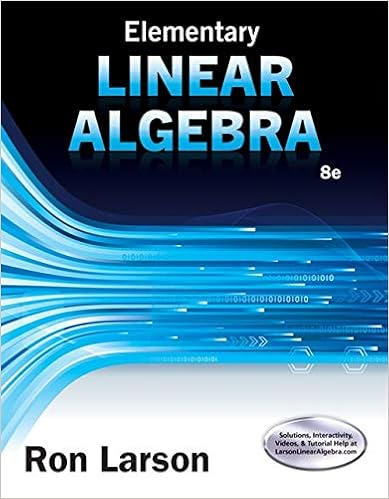# What is the basic difference between scalars and vectors? 2...

• Notes
• 4
• 50% (6) 3 out of 6 people found this document helpful

This preview shows page 1 - 2 out of 4 pages.

##### We have textbook solutions for you!
The document you are viewing contains questions related to this textbook.The document you are viewing contains questions related to this textbook.
Chapter 4 / Exercise 60
Elementary Linear Algebra
LarsonExpert Verified
Experiment 2 Objective 1. Obtain the resultant of a number of forces acting on a body. 2. Obtain the resultant of the same forces by drawing a vector diagram to scale to find its magnitude and direction. 3. Determine of the resultant using the analytical method, including the law of cosine for the magnitude and the law of sine to find its direction in one instance. The components method will also be used. MATERIALS 1. Force table 5. Strings for suspending the masses 2. Weight holders (#4). 6. A ring 3. Pulleys (#4). 7. A metal pin 4. Slotted weights 8. A protractor 9. Sheets of plain paper and graph paper. Review Questions and Exercises 1) What is the basic difference between scalars and vectors? 2) Is temperature is a vector quantity? What do plus and minus temperature signify? Explain. 3) A vector A has a magnitude of 60 m and directed as shown. Find the x and y components of this vector. y x 30 A 4) What are, briefly, the steps to find the resultant of vectors? 5) What is the difference between a resultant & equilibrant vector & what do they physically mean?
##### We have textbook solutions for you!
The document you are viewing contains questions related to this textbook.The document you are viewing contains questions related to this textbook.
Chapter 4 / Exercise 60
Elementary Linear Algebra
LarsonExpert Verified
•••